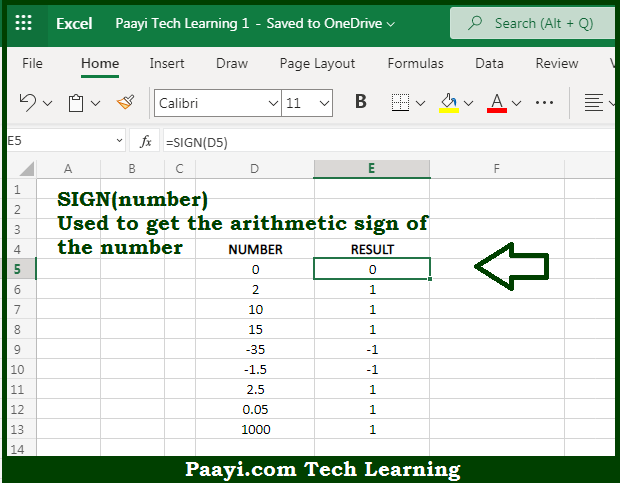# Learn How to Use Microsoft Excel SIGN Function

Written by | 0 Comments | 513 Views

In this article, you will learn how to use the Microsoft Excel SIGN function and its prime function in Microsoft Excel. You will also get to know the Microsoft Excel SIGN function return value and syntax with the help of some examples.

Microsoft Excel SIGN Function

The main function of the Microsoft Excel SIGN function is to get the sign of a given number. That implies, with the help of the SIGN function you can able to return the sign of the number in the form of +1, -1, or 0. It should be noted that if the number is positive, the SIGN function returns 1. If the number is negative, the SIGN function returns -1. If the number is zero, SIGN returns 0. So, with the help of the SIGN function, you can able to get the sign of the number in the form of +1, -1, or 0.

Return Value of SIGN Function

The return value will be +1 if positive, -1 if negative and 0 if zero.

Syntax of SIGN Function

=SIGN(number)

Where the arguments:

• number: This is the number of which you want to get the sign.

## How to Use Microsoft Excel SIGN Function?So we know that Microsoft Excel SIGN function you can able to get the sign of a given number. That implies, with the help of the SIGN function you can able to return the sign of the number in the form of +1, -1, or 0. It should be noted that if the number is positive, the SIGN function returns 1. If the number is negative, the SIGN function returns -1. If the number is zero, SIGN returns 0. So, with the help of the SIGN function, you can able to get the sign of the number in the form of +1, -1, or 0.